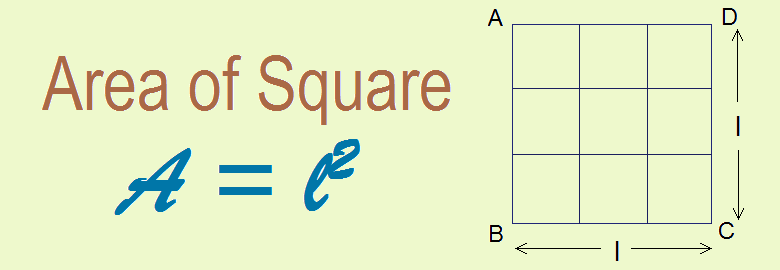# Area of Square## Area of Square

Area is the region covered by the plane figure. A square is a four sided plane figure having its all sides equal and each angle 90°. Area of square is measured by the product of its length and length i.e. length square.
Let ABCD be a square and it is divided into unit squares of side 1cm each and hence the area of each square is 1 cm2.
Now,
Area of the square ABCD = Total number of square boxes within the square ABCD.
= No. of squares along horizontal length × No. of squares along vertical breadth
= 3 × 3
= 9
Since each square box have the area 1 cm2
Area of the square ABCD = 9 × 1 cm2
= 9 cm2
Area of a square = length (l) × length (l) = l × l = l2

### Area of square in terms of diagonal

Diagonals of a square are equal and they bisect to each other at 90°. Let ABCD be the square and AC and BD are diagonals (d). Since diagonals are equal and they bisect to each other at 90°

Note: Area is measured in square units. For example cm2, mm2, m2 etc. are the units of area.

### Workout Examples

Example 1: Find the area of the given plane figure.
Solution: Here,
The given figure the square of length(l) = 6cm
Area of square = l2
= (6cm)2
= 36cm2

Example 2: Find the area of the given square.

Example 3: If the perimeter of a square garden is 160m, find its area and cost of paving its floor at Rs 200 per square meter.
Solution: Here,
Perimeter of square garden = 160m
i.e.          4l = 160m
or,           l = 160/4
or,          l = 40m

∴  Area of square = l2
= (40m)2
= 1600m2

Rate of paving floor = Rs. 200/m2

∴  Cost of paving = Rate × Area
= Rs. 200 × 1600
= Rs. 320000

You can comment your questions or problems regarding the area of square here.